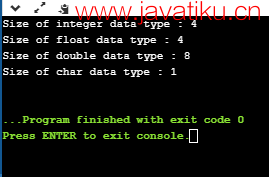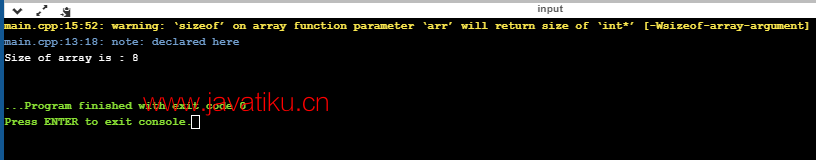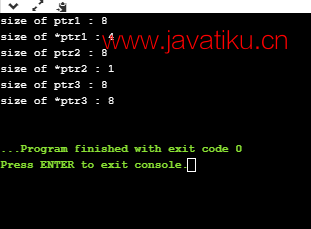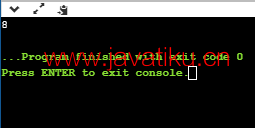# C++教程-C++中的sizeof()运算符## C++中的sizeof()运算符

sizeof()是一个运算符，用于计算数据类型、常量和变量的大小。它是一个编译时运算符，因为它在编译时返回变量或常量的大小。

sizeof()运算符计算出的大小是计算机中占用的RAM空间大小。

sizeof()运算符的语法如下：

``sizeof(数据类型); ``

sizeof()运算符可以应用于以下操作数类型：

• 当操作数为数据类型时

``````#include <iostream>
using namespace std;
int main()
{
// 确定每种数据类型占用的字节数。
std::cout << "整型数据类型的大小：" <<sizeof(int)<< std::endl;
std::cout << "浮点型数据类型的大小：" <<sizeof(float)<< std::endl;
std::cout << "双精度浮点型数据类型的大小：" <<sizeof(double)<< std::endl;
std::cout << "字符型数据类型的大小：" <<sizeof(char)<< std::endl;
return 0;
}``````• 当操作数为类类型时
``````#include <iostream>
using namespace std;
class Base
{
int a;
};
int main()
{
Base b;
std::cout << "类Base的大小为：" <<sizeof(b) << std::endl;
return 0;
}````````````#include <iostream>
using namespace std;
class Base
{
int a;
int d;
};
int main()
{
Base b;
std::cout << "类Base的大小为：" <<sizeof(b) << std::endl;
return 0;
}````````````#include <iostream>

using namespace std;

class Base
{
int a;
int d;
char ch;
};
int main()
{
Base b;
std::cout << "类Base的大小为：" <<sizeof(b) << std::endl;
return 0;
}``````• 当操作数为数组类型时
``````#include <iostream>
using namespace std;
int main()
{
int arr[]={10,20,30,40,50};
std::cout << "数组'arr'的大小为：" <<sizeof(arr) << std::endl;
return 0;
}````````````#include <iostream>
using namespace std;
void fun(int arr[])
{
std::cout << "数组的大小为：" <<sizeof(arr)<< std::endl;
}
int main()
{
int arr[]={10,20,30,40,50};
fun(arr);
return 0;
}``````• 当操作数为指针类型时
``````#include <iostream>
using namespace std;
int main()
{
int *ptr1=new int(10);
std::cout << "ptr1的大小：" <<sizeof(ptr1)<< std::endl;
std::cout << "*ptr1的大小：" <<sizeof(*ptr1)<< std::endl;
char *ptr2=new char('a');
std::cout <<"ptr2的大小：" <<sizeof(ptr2)<< std::endl;
std::cout <<"*ptr2的大小：" <<sizeof(*ptr2)<< std::endl;
double *ptr3=new double(12.78);
std::cout <<"ptr3的大小：" <<sizeof(ptr3)<< std::endl;
std::cout <<"*ptr3的大小：" <<sizeof(*ptr3)<< std::endl;
return 0;
}``````• 当操作数为表达式时
``````#include <iostream>
using namespace std;

int main()
{
int num1;
double num2;
cout << sizeof(num1+num2);
return 0;
}  ``````